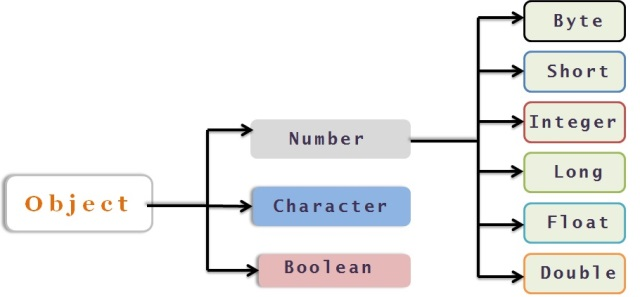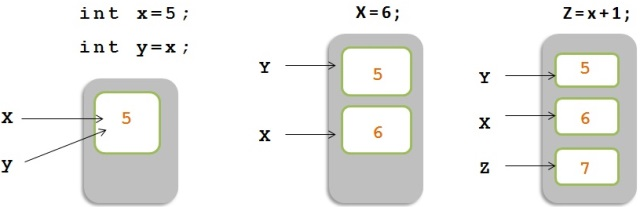# Wrapper class in Java

### Let us understand about Wrapper Class

We know that there are `8` primitive data types provided in java(like int,float ,boolean etc)

Java has provided the dedicated class for each of these primitive types and these classes are called as Wrapper class in Java.

They are called wrapper class as they wrap the primitive into an object.

Below table displays the primitive and corresponding wrapper class

Primitive TypeWrapper Class
booleanBoolean
byteByte
charCharacter
intInteger
floatFloat
doubleDouble
longLong
shortShort

##### Wrapper class Hierarchy###### Example:

1. int age=20; // defining “age” as primitive type
2. Integer id = new Integer(30); // defining “id” as a wrapper type “Integer”
```int age=20; // defining “age” as primitive type
Integer id = new Integer(30); // defining “id” as a wrapper type “Integer”```

`All the wrapper classes except Character wrapper class takes String as a parameter in the constructor.`

So we can write as below

1. Integer id= new Integer(30);
`Integer id= new Integer(“30”);`

Here, we need to pass string containing numbers only, if we pass string containing characters then it will throw an exception

###### Example

1. Integer id = new Integer(“Ten”);
`Integer id = new Integer(“Ten”);`

The above line will throw “ NumberFormatException ” as we need to pass only numbers.

We can convert wrapper object to primitive and primitive to wrapper object.

`The process of converting primitive to wrapper is called as “ Boxing ”.`
`The process of converting wrapper to primitive is called as “ Un-boxing ”`

Since boxing and un-boxing features converts primitive into object and object into primitive automatically and hence its named as Auto boxing and Auto un-boxing.

###### Example for Boxing

1. public class WrapperBoxing{
2. public static void main(String args[]){
3. //Converting int into Integer
4. int age=25;
5. Integer ageObj=Integer.valueOf(age);//converting int into Integer explicitly - Boxing
6. Integer ageObj1=age;//autoboxing, now compiler will add Integer.valueOf(age) automatically  - Auto boxing
7.
8. System.out.println(age+" "+ageObj+" "+ageObj1);
9. }
10. }
```public class WrapperBoxing{
public static void main(String args[]){
//Converting int into Integer
int age=25;
Integer ageObj=Integer.valueOf(age);//converting int into Integer explicitly - Boxing
Integer ageObj1=age;//autoboxing, now compiler will add Integer.valueOf(age) automatically  - Auto boxing

System.out.println(age+" "+ageObj+" "+ageObj1);
}
}  ```

###### Example for Unboxing

1. public class WrapperUnboxing {
2. public static void main(String args[]){
3. //Converting Integer to int
4. Integer ageObj=new Integer(25);
5. int age1=ageObj.intValue();//converting Integer to int  explicitly - unboxing
6. int age2=ageObj;//unboxing, now compiler will add  ageObj.intValue() automatically     - Auto unboxing
7.
8. System.out.println(ageObj+" "+age1+" "+age2);
9. }
10. }
```public class WrapperUnboxing {
public static void main(String args[]){
//Converting Integer to int
Integer ageObj=new Integer(25);
int age1=ageObj.intValue();//converting Integer to int  explicitly - unboxing
int age2=ageObj;//unboxing, now compiler will add  ageObj.intValue() automatically     - Auto unboxing

System.out.println(ageObj+" "+age1+" "+age2);
}
}```

Each wrapper class has lot of utility methods to convert primitive value to String and String to respective primitive value

##### Why do we need wrapper class when we already have primitive types?

In some cases, we can’t use primitives,

Example : If we want to add few integers to collection, we can’t use int primitives as collection takes only objects.

1. ArrayList<Integer> al = new ArrayList<Integer>(); //Valid
2. ArrayList<int> al = new ArrayList<int>(); //Invalid
```ArrayList<Integer> al = new ArrayList<Integer>(); //Valid
ArrayList<int> al = new ArrayList<int>(); //Invalid```

The wrapper classes provide many utility methods also like converting String number to integer and vice versa and converting number from decimal to binary and octal etc.

```Note :
All wrapper classes are immutable```

Immutable means that the object state can’t be changed for any modification.

If we modify anything, then new object will be created without affecting existing object.

###### Example:

1. Integer x = new Integer(5);//assign new integer to x
2. Integer y = x;//y refences same integer as x
3. x= 6;//modify x with new value
4. z=x+1;
5. System.out.println(x);
6. System.out.println(y);
7. System.out.println(z);
```Integer x = new Integer(5);//assign new integer to x
Integer y = x;//y refences same integer as x
x= 6;//modify x with new value
z=x+1;
System.out.println(x);
System.out.println(y);
System.out.println(z);```If Integer wrapper would have been mutable, then we would have got output as